# Rectangular Contracted Weir

This calculates the water flow rate over a rectangular contracted weir. This weir has a rectangular opening where the sides are straight up and down. A contracted weir means that the ditch or canal leading up to the weir is wider than the weir opening itself. The water before the weir should be held in a relatively calm and smooth pool. There should be air (not trapped) underneath the water leaving the weir. The Length is the bottom width of the weir. The height is measured from the bottom of the weir opening to the top of the water level ponded behind the weir (not the water level right as it leaves the weir). Learn more about the units used on this page.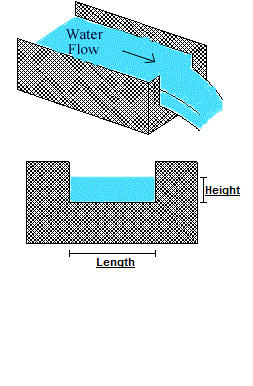Length:
Height:
Flow Rate:

* Note: 1 point = 1/100 ft.

## The Equation

The Equation used to determine the flow rate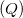of a Rectangular Contracted Weir is: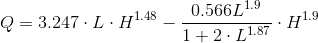Where: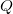= Flow Rate in cfs.= Bottom width of the weir in feet.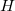= Height of the upstream water above the weir crest in feet.

WSU Prosser - IAREC, 24106 N Bunn Rd, Prosser WA 99350-8694, 509-786-2226. Contact Us